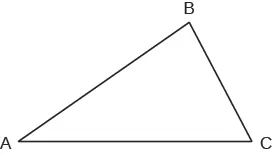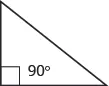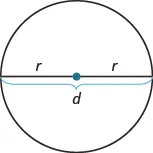Prealgebra

# Key Concepts

PrealgebraKey Concepts

### Key Concepts

#### 9.1Use a Problem Solving Strategy

• Problem Solving Strategy
1. Step 1. Read the word problem. Make sure you understand all the words and ideas. You may need to read the problem two or more times. If there are words you don't understand, look them up in a dictionary or on the internet.
2. Step 2. Identify what you are looking for.
3. Step 3. Name what you are looking for. Choose a variable to represent that quantity.
4. Step 4. Translate into an equation. It may be helpful to first restate the problem in one sentence before translating.
5. Step 5. Solve the equation using good algebra techniques.
6. Step 6. Check the answer in the problem. Make sure it makes sense.
7. Step 7. Answer the question with a complete sentence.

#### 9.2Solve Money Applications

• Finding the Total Value for Coins of the Same Type
• For coins of the same type, the total value can be found as follows:
$number·value=total valuenumber·value=total value$
where number is the number of coins, value is the value of each coin, and total value is the total value of all the coins.
• Solve a Coin Word Problem
1. Step 1. Read the problem. Make sure you understand all the words and ideas, and create a table to organize the information.
2. Step 2. Identify what you are looking for.
3. Step 3.
Name what you are looking for. Choose a variable to represent that quantity.
• Use variable expressions to represent the number of each type of coin and write them in the table.
• Multiply the number times the value to get the total value of each type of coin.
4. Step 4. Translate into an equation. Write the equation by adding the total values of all the types of coins.
5. Step 5. Solve the equation using good algebra techniques.
6. Step 6. Check the answer in the problem and make sure it makes sense.
7. Step 7. Answer the question with a complete sentence.
• Type $NumberNumber$ $Value ()Value ()$ $Total Value ()Total Value ()$

#### 9.3Use Properties of Angles, Triangles, and the Pythagorean Theorem

• Supplementary and Complementary Angles
• If the sum of the measures of two angles is 180°, then the angles are supplementary.
• If $∠A∠A$ and $∠B∠B$ are supplementary, then $m∠A+m∠B=180m∠A+m∠B=180$.
• If the sum of the measures of two angles is 90°, then the angles are complementary.
• If $∠A∠A$ and $∠B∠B$ are complementary, then $m∠A+m∠B=90m∠A+m∠B=90$.
• Solve Geometry Applications
1. Step 1. Read the problem and make sure you understand all the words and ideas. Draw a figure and label it with the given information.
2. Step 2. Identify what you are looking for.
3. Step 3. Name what you are looking for and choose a variable to represent it.
4. Step 4. Translate into an equation by writing the appropriate formula or model for the situation. Substitute in the given information.
5. Step 5. Solve the equation using good algebra techniques.
6. Step 6. Check the answer in the problem and make sure it makes sense.
7. Step 7. Answer the question with a complete sentence.
• Sum of the Measures of the Angles of a Triangle• For any $ΔABC,ΔABC,$ the sum of the measures is 180°
• $m∠A+m∠B=180m∠A+m∠B=180$
• Right Triangle• A right triangle is a triangle that has one 90° angle, which is often marked with a $⦜⦜$symbol.
• Properties of Similar Triangles
• If two triangles are similar, then their corresponding angle measures are equal and their corresponding side lengths have the same ratio.

#### 9.4Use Properties of Rectangles, Triangles, and Trapezoids

• Properties of Rectangles
• Rectangles have four sides and four right (90°) angles.
• The lengths of opposite sides are equal.
• The perimeter, $PP$, of a rectangle is the sum of twice the length and twice the width.
• $P=2L+2WP=2L+2W$
• The area, $AA$, of a rectangle is the length times the width.
• $A=L⋅WA=L⋅W$
• Triangle Properties
• For any triangle $ΔABCΔABC$, the sum of the measures of the angles is 180°.
• $m∠A+m∠B+m∠C=180°m∠A+m∠B+m∠C=180°$
• The perimeter of a triangle is the sum of the lengths of the sides.
• $P=a+b+cP=a+b+c$
• The area of a triangle is one-half the base, b, times the height, h.
• $A=12bhA=12bh$

#### 9.5Solve Geometry Applications: Circles and Irregular Figures

• Problem Solving Strategy for Geometry Applications
1. Step 1. Read the problem and make sure you understand all the words and ideas. Draw the figure and label it with the given information.
2. Step 2. Identify what you are looking for.
3. Step 3. Name what you are looking for. Choose a variable to represent that quantity.
4. Step 4. Translate into an equation by writing the appropriate formula or model for the situation. Substitute in the given information.
5. Step 5. Solve the equation using good algebra techniques.
6. Step 6. Check the answer in the problem and make sure it makes sense.
7. Step 7. Answer the question with a complete sentence.
• Properties of Circles• $d=2rd=2r$
• Circumference: $C=2πrC=2πr$ or $C=πdC=πd$
• Area: $A=πr2A=πr2$

#### 9.6Solve Geometry Applications: Volume and Surface Area

• Volume and Surface Area of a Rectangular Solid
• $V=LWHV=LWH$
• $S=2LH+2LW+2WHS=2LH+2LW+2WH$
• Volume and Surface Area of a Cube
• $V=s3V=s3$
• $S=6s2S=6s2$
• Volume and Surface Area of a Sphere
• $V=43πr3V=43πr3$
• $S=4πr2S=4πr2$
• Volume and Surface Area of a Cylinder
• $V=πr2hV=πr2h$
• $S=2πr2+2πrhS=2πr2+2πrh$
• Volume of a Cone
• For a cone with radius $rr$ and height $hh$:
Volume: $V=13πr2hV=13πr2h$

#### 9.7Solve a Formula for a Specific Variable

• Distance, Rate, and Time
• $d=rtd=rt$
Order a print copy

As an Amazon Associate we earn from qualifying purchases.